• 最大似然参数估计的基本原理
千次阅读
2021-09-19 13:16:45

# 最大似然参数估计的基本原理

前导知识：【概率密度函数估计的引入】

在最大似然估计中，我们做以下基本假设：

• 我们把要估计的参数记作 θ \theta ，它是确定但未知的量（多个参数时向量）。
• 每类的样本集记作 X i , i = 1 , 2 , . . . , c X_i,i=1,2,...,c ，其中的样本都是从密度为 ρ ( x ∣ w i ) \rho(x|w_i) 的总体中独立抽取出来的，即所谓满足独立同分布条件。
• 类条件概率密度函数 ρ ( x ∣ w i ) \rho(x|w_i) 具有确定函数形式，只是其中的参数 θ \theta 未知。比如在 x x 是一维正态分布 N ( μ , σ 2 ) N(\mu,\sigma^2) 时，未知参数可能是 θ = [ μ , σ 2 ] T \theta=[\mu,\sigma^2]^T ，对不同类别的参数可以记作 θ i \theta_i ，为了强调概率密度中待估计的参数，可以把 ρ ( x ∣ w i ) \rho(x|w_i) 写作 ρ ( x ∣ w i , θ i ) \rho(x|w_i,\theta_i) ρ ( x ∣ t h e t a i ) \rho(x|theta_i)
• 各类样本只包含本类的分布信息，即：不同类别的参数是独立的。可以分别对每一类单独处理。

在上述假设前提下，可以分别处理 c c 个独立的问题。即，在一类中独立地按照概率密度 ρ ( x ∣ θ ) \rho(x|\theta) 抽取样本集 X X ，用 X X 来估计出未知参数 θ \theta

设样本集包含 N N 个样本，即：
X = { x 1 , x 2 , . . . , x N } (1) X=\{x_1,x_2,...,x_N\} \tag 1
由于样本是独立地从 ρ ( x ∣ θ ) \rho(x|\theta) 中抽取的，所以在概率密度为 ρ ( x ∣ θ ) \rho(x|\theta) 时获得样本集 X X 的概率即出现 X X 中的各个样本的联合概率是：
l ( θ ) = ρ ( X ∣ θ ) = ρ ( x 1 , x 2 , . . . , x N ∣ θ ) = ∏ i = 1 N ρ ( x i ∣ θ ) (2) l(\theta)=\rho(X|\theta)=\rho(x_1,x_2,...,x_N|\theta)=\prod_{i=1}^{N} \rho(x_i|\theta) \tag 2
这个概率反映了在概率密度函数的参数是 θ \theta 时，得到式 ( 1 ) (1) 中这组样本的概率。因为样本集已知，而参数 θ \theta 未知，式 ( 2 ) (2) 就成为了 θ \theta 的函数，它反映的是在不同参数取值下取得当前样本集的可能性，因此称作参数 θ \theta 相对于样本集 X X 的似然函数，式 ( 2 ) (2) 中每一项 ρ ( x i ∣ θ ) \rho(x_i|\theta) 就是 θ \theta 相对于每一个样本的似然函数。
似然函数 l ( θ ) l(\theta) 给出了从总体中抽取 x 1 , x 2 , . . . , x N x_1,x_2,...,x_N 这样 N N 个样本的概率。即，参数估计的过程即在参数空间中寻找使得似然函数 l ( θ ) l(\theta) 最大的那个 θ \theta
一般来说，使似然函数的值最大的 θ ^ \hat{\theta} 的样本 x 1 , x 2 , . . . , x N x_1,x_2,...,x_N 的函数，记为：
θ ^ = d ( x 1 , x 2 , . . . , x N ) (3) \hat{\theta}=d(x_1,x_2,...,x_N) \tag 3
此时称 θ ^ \hat{\theta} 叫做 θ \theta 的最大似然估计量。
下面给出 N = 1 N=1 x x 为一维且具有以均值为3，方差为1的正态分布（那么此时“最可能出现的”样本就是 x 1 ′ = 3 x_1'=3 ）：
θ \theta 在参数空间中取不同值时：（观察图像可以取定 x = 3 x=3当固定 x = 3 x=3 时：（观察图像令参数取不同值）最大似然估计量：令 l ( θ ) l(\theta) 为样本集 X X 的似然函数， X = { x 1 , x 2 , . . . , x N } X=\{x_1,x_2,...,x_N\} ，如果 θ ^ = d ( X ) = d ( x 1 , x 2 , . . . , x N ) \hat{\theta}=d(X)=d(x_1,x_2,...,x_N) 是参数空间中能使似然函数 l ( θ ) l(\theta) 极大化的 θ \theta 值，那么 θ ^ \hat{\theta} 就是 θ \theta 的最大似然估计量，或者记作：
θ ^ = a r g   m a x   l ( θ ) (4) \hat{\theta} = arg \ max \ l(\theta) \tag 4
其中， a r g   m a x arg \ max 是一种常用的表示方法，表示使后面的函数取得最大值的变量的取值。为了便于分析，还可以定义对数似然函数：
H ( θ ) = ln ⁡ l ( θ ) = ln ⁡ ∏ i = 1 N ρ ( x i ∣ θ ) = ∑ i = 1 N ln ⁡ ρ ( x i ∣ θ ) (5) H(\theta)=\ln l(\theta)=\ln \prod_{i=1}^{N} \rho(x_i|\theta) =\sum_{i=1}^{N} \ln \rho(x_i|\theta) \tag 5
容易证明，使对数似然函数最大的 θ \theta 值也使似然函数最大。参数空间 类条件概率密度
更多相关内容
• 这是模式分类中的课程代码， ...对不同维数下的高斯概率密度模型，用最大似然估计方法对其参数进行估计，学习和掌握最 大似然估计方法。 (a) 编写程序，对表格2 中的类1 w 中的3 个特征i x ，分别求解最大似然估计μ
• 该文将马尔可夫链蒙特卡洛 (Markov chain MonteCarlo, MCMC)方法和均值似然估计相结合,利用离散调频图(chirpogram)作为起始点的选择方法,提出了一种实现单分量 chirp信号最大似然参数估计的新方法。仿真和分析表明...
• 最小二乘法曲线拟合参数估计：简单起见，这里以一元线性回归为例进行介绍：假设我们获取了一组样本点数据：利用最小二乘法用多项式曲线拟合这组样本点:1、设拟合多项式为：2、样本点到该曲线的距离平方和为：目标...

最小二乘法曲线拟合参数估计：

简单起见，这里以一元线性回归为例进行介绍：

假设我们获取了一组样本点数据：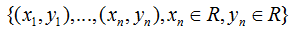利用最小二乘法用多项式曲线拟合这组样本点:

1、设拟合多项式为：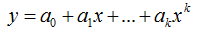2、样本点到该曲线的距离平方和为：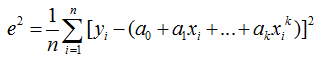目标函数为：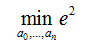上式对参数求偏导有：则上式等价于：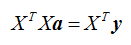若X’X的逆矩阵存在：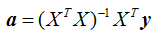是一个解析解(即一步到位的解法)

在测试中，我们假设训练样本是在曲线y=(x2-1)3+(x-0.5)2+3sin(2x)的基础上对x和y做随机拉伸处理生成的，我们假设拟合多项式阶数为9(可以用交叉验证来获取)，用上述方法拟合得到的结果如下图所示：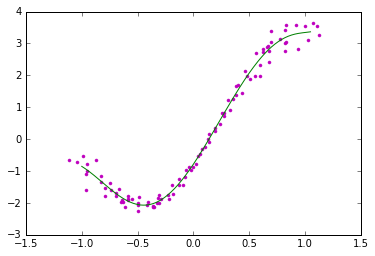Python代码如下：

import numpy as np

import random

import matplotlib.pyplot as plt

fig = plt.figure()

#阶数为9

order = 9

#生成样本点

x = np.arange(-1,1,0.02)

y = [((a*a-1)**3 + (a-0.5)**2 + 3*np.sin(2*a)) for a in x]

#ax.plot(x,y,color='r',linestyle='-',marker='')

x_a = [b1*(random.randint(90,120))/100 for b1 in x]

y_a = [b2*(random.randint(90,120))/100 for b2 in y]

ax.plot(x_a,y_a,color='m',linestyle='',marker='.')

#曲线拟合

#创建矩阵

#初始化而为数组

array_x =[[0 for i in range(order+1)] for i in range(len(x_a))]

#对二维数组赋值

for i in range(0,order+1):

for j in range(0,len(x_a)):

array_x[j][i] = x_a[j]**i

#将赋值后的二维数组转化为矩阵

matx=np.matrix(array_x)

matrix_A = matx.T*matx

yy = np.matrix(np.array(y_a))

matrix_B = matx.T*yy.T

matAA = np.linalg.solve(matrix_A,matrix_B).tolist()

#画出拟合后的曲线

xxa = np.arange(-1,1.06,0.01)

yya = []

for i in range(0,len(xxa)):

yyy=0.0

for j in range(0,order+1):

dy = 1.0

for k in range(0,j):

dy*=xxa[i]

dy*=matAA[j]

yyy+=dy

yya.append(yyy)

ax.plot(xxa,yya,color='g',linestyle='-',marker='')

ax.legend()

plt.show()

多元线性回归中参数的最大似然估计：

假设有m个训练样本(x, y)：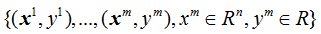假定预测值与样本特征间的函数关系是线性的，即线性回归分析，就在于根据样本x和y的观察值，去估计函数h(x)，定义为：1、对于第个训练样本，假设待估计函数h(x)与真实值y存在误差如下式所示：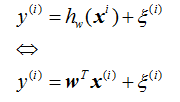假设：误差(即噪声)服从标准正太分布，则有：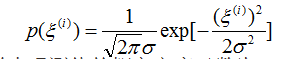2、单个样本下的组样本观测值的概率密度函数为：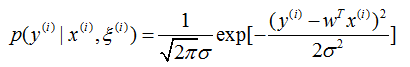3、根据最大似然概率的准则可得似然函数如下：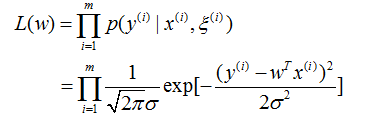目标函数为：max(L(w))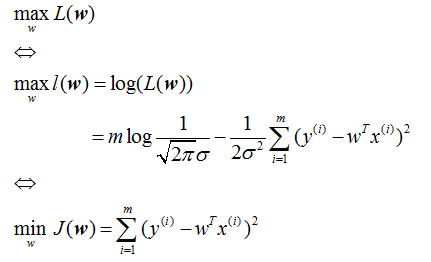4、同第一部分，对上式求偏导数并令其等于0可得：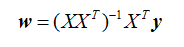上式只在逆矩阵存在的时候适用。

在测试中，假设样本生成式为:y=0.5x+4*randuniform(0,1)*sin(2x)+3.5，用上述方法做多元线性回归得到的结果下图所示：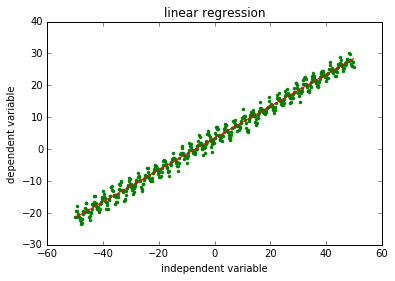Python代码如下：

import numpy as np

import random

import matplotlib.pyplot as plt

#生成样本点

x = np.arange(-50,50,0.2)

array_x = []

array_y = []

for a in x:

lineX = 

lineX.append(a)

array_x.append(lineX)

array_y.append(0.5*a + 3.5 + random.uniform(0,1)*4*np.sin(2*a))

#线性回归

xMat = np.mat(array_x)

yMat = np.mat(array_y).T

xTx = xMat.T*xMat

w = xTx.I*xMat.T*yMat

y = xMat*w

#画图

plt.title("linear regression")

plt.xlabel("independent variable")

plt.ylabel("dependent variable")

plt.plot(x,array_y,color='g',linestyle='',marker='.')

plt.plot(x,y,color='r',linestyle='-',marker='')

plt.show()

展开全文•matlab
• 主要介绍了python简单实现最大似然估计&scipy库的使用详解，具有很好的参考价值，希望对大家有所帮助。一起跟随小编过来看看吧
• 最大似然估计，通俗说，利用已知的样本结果，反推最有可能（最大概率）导致这样结果的参数似然也是用于表征概率的，只不过这个概率是我们已经知道事件的结果，而去反推事件发生环境的参数的概率，我们认为事件是...

最大似然估计，通俗说，利用已知的样本结果反推最有可能（最大概率）导致这样结果的参数值

似然也是用于表征概率的，只不过这个概率是我们已经知道事件的结果，而去反推事件发生环境的参数的概率，我们认为事件是在最可能发生该事件的环境参数下发生的，有点绕

举个例子：抛硬币，假设我们随机抛掷一枚硬币1,000次，结果500次人头朝上，500次数字朝上，不管怎样，我们现在就是有着这么一个perfect结果，我们现在就很好奇，到底是什么导致了这么完美的结果，于是我们就要反推做这个实验时的涉及到的参数，我们认为所有的一切都不可能是巧合，只有各种条件都是满足的，才有可能发生这样完美的1/2的概率，此时1/2的概率是作为结果的，因为我们做实验得出了这样的结果啊。经过我们计算发现，一枚标准的硬币，使得发生了【两面朝上的概率均为50%】这个事件的可能性最大；但是，如果是不标准的硬币，随便抛，能不能得出正反面各50%的频率这个结果呢，想想这也是有可能的嘛；所以我们只能说，如果这个硬币是标准硬币，发生正反面各50%的概率是最大的，因为这样子搞可以最大可能的出现我们期待的结果。我们运用出现的结果来判断这个事情本身的性质（参数），就是似然。 似然描述的是结果已知的情况下，该事件在不同条件下发生的可能性，似然函数的值越大说明该事件在对应的条件下发生的可能性越大

再来个例子：有两个妈妈带着一个小孩到了你的面前，妈妈A和小孩长得很像，妈妈B和小孩一点都不像，问你谁是孩子的妈妈，你说是妈妈A。好的，那这种时候你所采取的方式就是极大似然估计：妈妈A和小孩长得像，所以妈妈A是小孩的妈妈的概率大，这样妈妈A看来就是小孩的妈妈，妈妈A就是小孩的妈妈。

极大似然估计就是在只有概率的情况下，忽略低概率事件直接将高概率事件认为是真实事件的思想。

结果和参数相互对应的时候，似然和概率在数值上是相等的，如果用 θ 表示环境对应的参数，x 表示结果，那么概率可以表示为：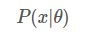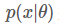是条件概率的表示方法，θ 是前置条件，在 θ 的前提下，事件 x 发生的概率，相对应的似然可以表示为：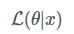可以理解为已知结果为 x ，参数为 θ 对应的概率，即：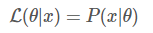需要说明的是两者在数值上相等，但是意义并不相同，L 是关于 θ 的函数，而 PP 则是关于 x 的函数，两者从不同的角度描述一件事情。

### 似然函数的最大值

似然函数的最大值意味着什么？

概率描述的是在一定条件下某个事件发生的可能性，概率越大说明这件事情越可能会发生；

似然描述的是结果已知的情况下，该事件在不同条件下发生的可能性，似然函数的值越大说明该事件在对应的条件下发生的可能性越大。

我们关注似然函数的最大值，因为我们需要根据已知事件来找出产生这种结果最有可能的条件，目的当然是根据这个最有可能的条件去推测未知事件的概率。在抛硬币的事件中，环境参数 p 可以取 [0, 1] 内的所有值，这是由硬币的性质所决定的，如果硬币均匀p=0.5，如果硬币不均匀则p就为其他值，显而易见，当 p=0.5 这种均匀硬币最有可能产生我们观测到的正反各为50%朝上。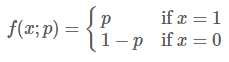似然函数的最大值意味着某个环境参数下最有可能发生我们观测到的事件，那我们就认为这个环境参数就是真实的环境参数，因为只有在这个环境参数下才能最大概率的发生我们观测到的结果，这样我们就可以根据似然函数通过求导算出极大值，计算出该极大值下的环境参数，然后我们就可以用这个环境参数正向计算其他事件发生的概率P(x|θ)

### 对数似然函数

实际问题往往会涉及到多个独立事件，在似然函数的表达式中通常都会出现连乘：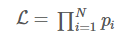对多项乘积的求导往往非常复杂，但是对于多项求和的求导却要简单的多，对数函数不改变原函数的单调性和极值位置，而且根据对数函数的性质可以将乘积转换为加减式，这可以大大简化求导的过程：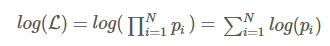例子：

假如有一个罐子，里面有黑白两种颜色的球，数目多少不知，两种颜色的比例也不知。我 们想知道罐中白球和黑球的比例，但我们不能把罐中的球全部拿出来数。现在我们可以每次任意从已经摇匀的罐中拿一个球出来，记录球的颜色，然后把拿出来的球 再放回罐中。这个过程可以重复，我们可以用记录的球的颜色来估计罐中黑白球的比例。假如在前面的一百次重复记录中，有七十次是白球，请问罐中白球所占的比例最有可能是多少？很多人马上就有答案了：70%。而其后的理论支撑是什么呢？

我们假设罐中白球的比例是p，那么黑球的比例就是1-p。因为每抽一个球出来，在记录颜色之后，我们把抽出的球放回了罐中并摇匀，所以每次抽出来的球的颜色服从同一独立分布。这里我们把一次抽出来球的颜色称为一次抽样。题目中在一百次抽样中，七十次是白球的概率是P(Data | M)，这里Data是所有的数据，M是所给出的模型，表示每次抽出来的球是白色的概率为p。如果第一抽样的结果记为x1，第二抽样的结果记为x2... 那么Data = (x1,x2,…,x100)。

P(Data | M)= P(x1,x2,…,x100|M)

= P(x1|M)P(x2|M)…P(x100|M)

= p^70(1-p)^30.

那么p在取什么值的时候，P(Data |M)的值最大呢？将p^70(1-p)^30对p求导，并其等于零。

70p^69(1-p)^30-p^70*30(1-p)^29=0。

解方程可以得到p=0.7。

所以当p=0.7时，P(Data|M)的值最大。这和我们常识中按抽样中的比例来计算的结果是一样的。

连续变量：

给定一堆数据，假如我们知道它是从某一种分布中随机取出来的，可是我们并不知道这个分布具体的参数，即“模型已定，参数未知”。例如，我们知道这个分布是正态分布，但是不知道均值和方差；或者是二项分布，但是不知道均值。 最大似然估计就可以用来估计模型的参数。极大似然估计的目标是找出一组参数，使得模型产生出观测数据的概率最大

即：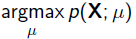我们假设每个观测数据是独立的，那么有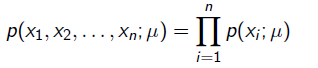为了求导方便，对目标取log，最优化似然函数等同于最优化对数似然函数：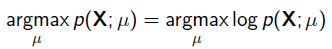### 最大似然估计小结

最大似然估计总是能精确地得到解吗？

简单来说，不能。在真实的场景中，更有可能的是，对数似然函数的导数仍然是难以解析的（也就是说，很难甚至不可能人工对函数求微分）。因此，一般采用期望最大化（EM）算法等迭代方法为参数估计找到数值解，但总体思路还是一样的。

为什么叫「最大似然（最大可能性）」，而不是「最大概率」呢？

大多数人倾向于混用「概率」和「似然度」这两个名词，但统计学家和概率理论家都会区分这两个概念。通过观察这个等式，我们可以更好地明确这种混淆的原因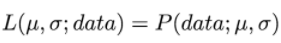这两个表达式是相等的！所以这是什么意思？我们先来定义 P(data; μ, σ) 它的意思是「在模型参数μ、σ条件下，观察到数据 data 的概率」。值得注意的是，我们可以将其推广到任意数量的参数和任何分布。

另一方面，L(μ, σ; data) 的意思是「我们在观察到一组数据 data 之后，参数μ、σ取特定的值的似然度。」

这个等式的含义就是：给定参数后数据的概率等于给定数据后参数的似然度。但是，尽管这两个值是相等的，但是似然度和概率从根本上是提出了两个不同的问题：一个是关于数据的，另一个是关于参数值的。这就是为什么这种方法被称为最大似然法（极大可能性），而不是最大概率。

展开全文• 转：http://blog.csdn.net/pipisorry/article/details/51461997最大似然估计MLE顾名思义...最大似然估计就是要用似然函数取到最大值时的参数值作为估计值，似然函数可以写做Note: p(x|theta)不总是代表条件概率；也...

# 最大似然估计MLE

顾名思义，当然是要找到一个参数，使得L最大，为什么要使得它最大呢，因为X都发生了，即基于一个参数发生的，那么当然就得使得它发生的概率最大。

最大似然估计就是要用似然函数取到最大值时的参数值作为估计值，似然函数可以写做

Note: p(x|theta)不总是代表条件概率；也就是说p(x|theta)不代表条件概率时与p(x;theta)等价，而一般地写竖杠表示条件概率，是随机变量；写分号p(x; theta)表示待估参数（是固定的，只是当前未知）,应该可以直接认为是p(x)，加了;是为了说明这里有个theta的参数，p(x; theta)意思是随机变量X=x的概率。在贝叶斯理论下又叫X=x的先验概率。相乘因为它们之间是独立同分布的。

MLE通常使用对数似然函数

使用log-likelihood比原始函数好的原因：

1 由于有连乘运算，通常对似然函数取对数计算简便，即对数似然函数。it's kind of analytically nice to work with log-likelihood.

2 multiplying small numbers the numerical errors start to add up and start to propagate.If we are summing together small numbers,the numerical errors are not so serious.

3 log函数是单调的，所有东西保持不变。

最大似然估计问题可以写成

这是一个关于的函数，求解这个优化问题通常对求导，得到导数为0的极值点。该函数取得最大值是对应的的取值就是我们估计的模型参数。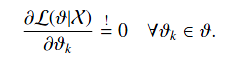### 给定观测到的样本数据，一个新的值发生的概率是

求出参数值不是最终目的，最终目的是去预测新事件基于这个参数下发生的概率。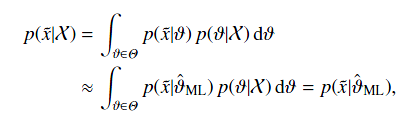Note: 注意有一个约等于，因为他进行了一个近似的替换，将theta替换成了估计的值，便于计算。that is, the next sample is anticipated to be distributed with the estimated parameters θ ˆ ML .

### 扔硬币的伯努利实验示例

以扔硬币的伯努利实验为例子，N次实验的结果服从二项分布，参数为P，即每次实验事件发生的概率，不妨设为是得到正面的概率。为了估计P，采用最大似然估计，似然函数可以写作

其中表示实验结果为i的次数。下面求似然函数的极值点，有

得到参数p的最大似然估计值为

可以看出二项分布中每次事件发的概率p就等于做N次独立重复随机试验中事件发生的概率。

如果我们做20次实验，出现正面12次，反面8次，那么根据最大似然估计得到参数值p为12/20 = 0.6。

### MLE的一个最简单清晰的示例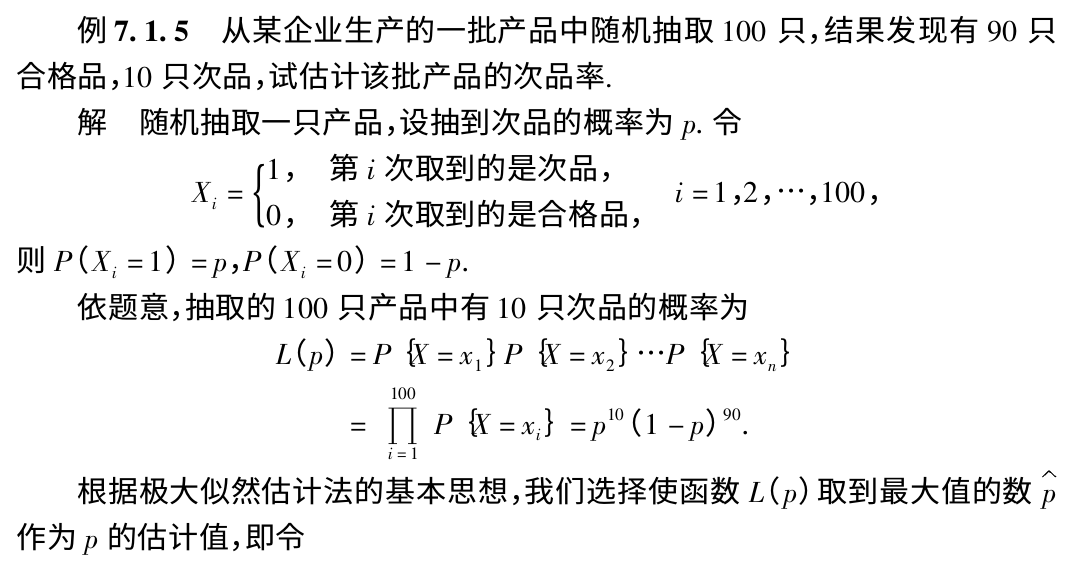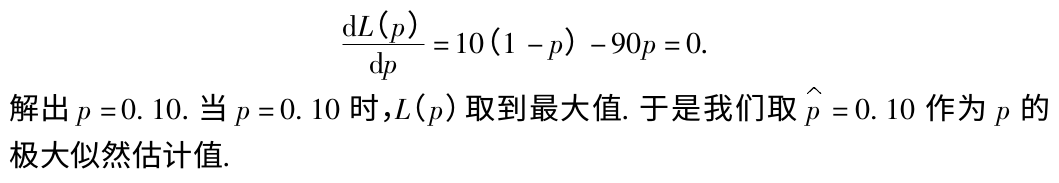皮皮blog

# 最大似然估计MLE

能最大化已观测到的观测序列的似然的参数就是估计的参数值。## 图钉的例子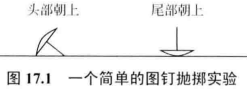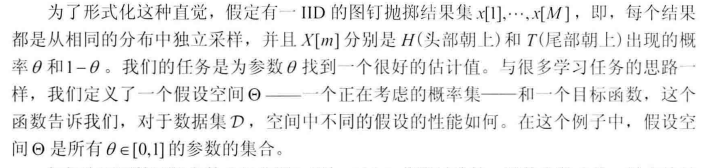### 为不同参数theta的可能值打分并选择的一种标准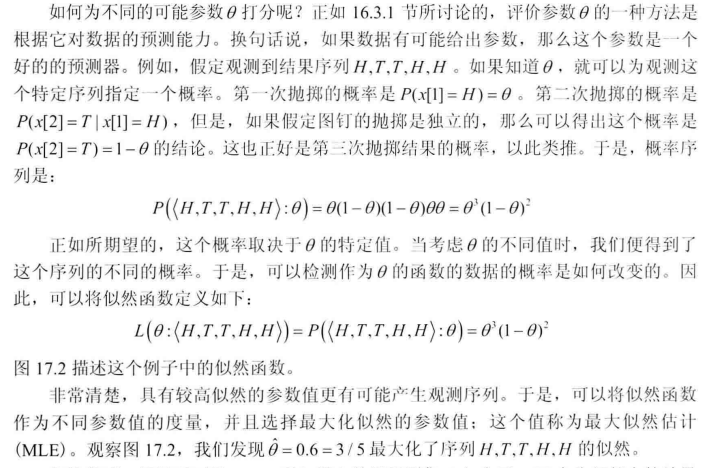### 一般情况下的MLE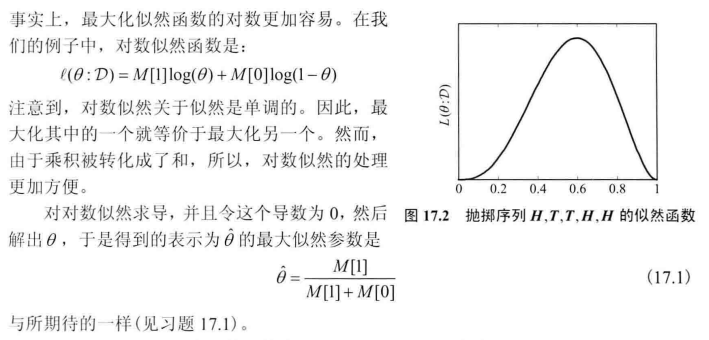## 最大似然准则

### 参数模型和参数空间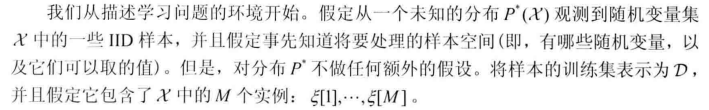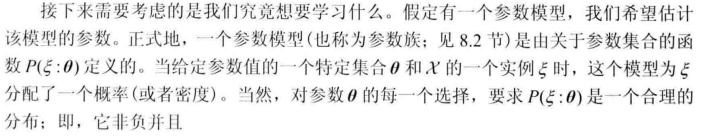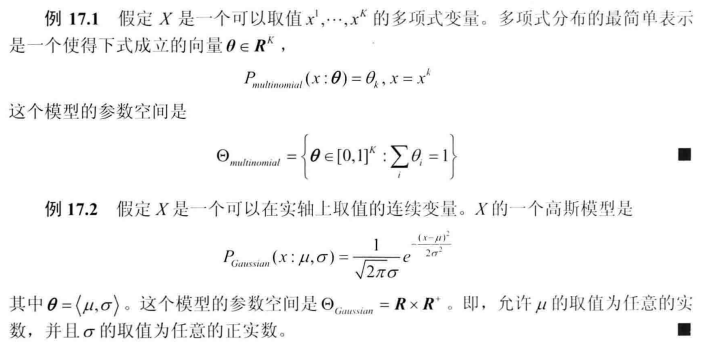### 似然函数的定义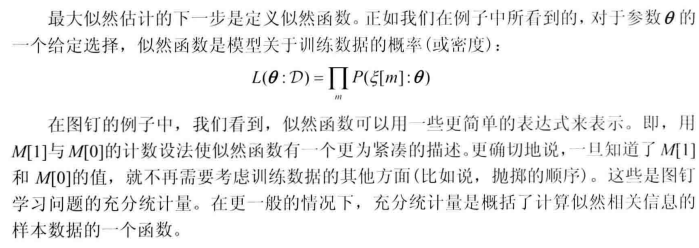充分统计量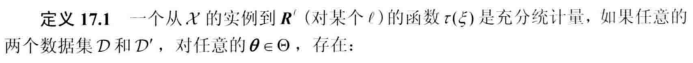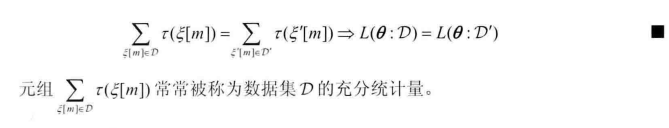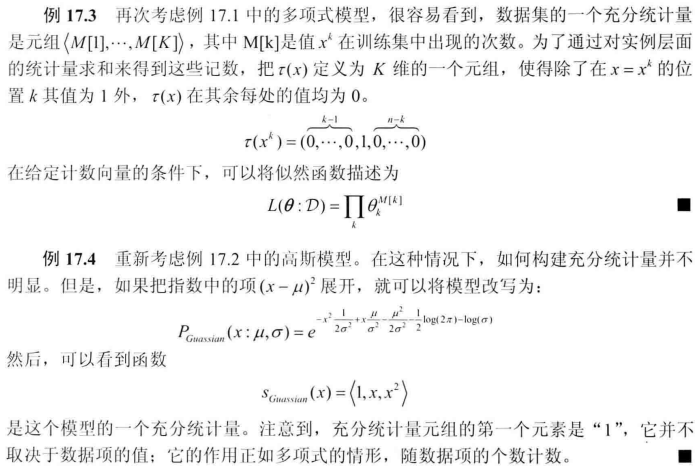### MLE的注解

MLE的缺陷：置信区间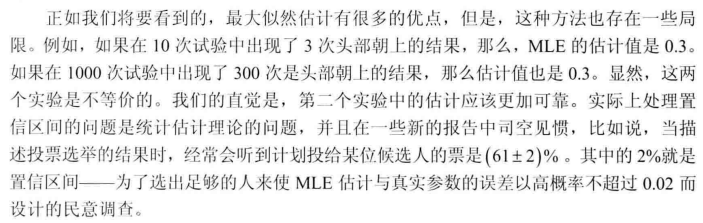似然函数度量了参数选择对于训练数据的影响。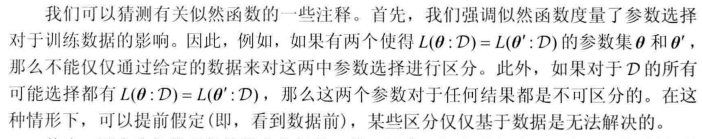似然函数的要求[《Probabilistic Graphical Models：Principles and Techniques》(简称PGM)]

展开全文• 对于给定的数据集，该方法寻找最有可能产生输出或最大似然函数的参数值。 最大似然估计估计一些问题提供了很好的方法，但在一些复杂的情况下它可能无法估计。 对数似然函数是似然函数的对数，对于通过将乘积项...
• ## 最大似然估计详解

千次阅读 2021-06-09 11:34:28
它是机器学习中常用的一种参数估计方法。它提供了一种给定观测数据来评估模型参数的方法。也就是模型已知，参数未定。   在我们正式讲解极大似然估计之前，我们先简单回顾以下两个概念： 概率密度函数...算法 机器学习
• 阅读【神经网络与机器学习】第二章的最大似然参数估计的感想。 书中具体内容请查看原文，大体内容就是讲最大似然参数估计算法，但其中有涉及到正则化。 其中的正则化项即是待估计参数W的先验概率，书中说W的先验...
• % ************************************************ % 一个数组信号参数最大似然估计问题% % 课程 EEL6537 的练习题和研究课题 12-02-22 孙磊 bitsunlei@126.com % 最后修改时间：2012 年 2 月 24 日% % 这是EEL...matlab
• 贝叶斯估计最大似然估计的原理及代码matlab贝叶斯
• 针对有色噪声干扰的多变量Hammerstein系统存在维数高和模型难以参数化的问题，本研究提出了递推极大似然参数估计方法，并对极大化似然函数系统参数向量的估计公式进行推导，同时给出了极大似然参数的辨识原理，并对...
• 本文的主要目的是利用归约技术研究参数估计方法的行为和性质。 我们将重点介绍一种使用修正得分函数来减少最大似然估计值偏差的方法。 我们还通过不同样本量和结果变量概率百分比的模拟数据提供了有趣的新示例。
• ## 极大似然估计和最大似然估计定义

万次阅读 多人点赞 2018-01-28 18:54:39
然后还有个最大似然估计，最要命的是我发现还有人专门对两者区别做了论述。然后我就看了下英文定义： 最大似然估计(maximum likelihood estimation, MLE) 极大似然估计方法（Maximum Likelihood Estimate，MLE...定义
• Matlab函数:极大似然估计 function [para,standard_deviation,fv]=my_mle(fun,para0,varargin) %estimate ......Matlab统计工具箱给出了常用概率分布中参数 的点估计 (采用最大似然估计法) 与区间估计, 另 外还提供...
• 本文考虑了有色噪声反馈非线性系统的参数估计。 根据数据滤波技术和最大似然原理，针对所讨论的反馈非线性系统，提出了一种最大似然遗忘因子随机梯度辨识方法。 仿真结果表明，该算法是有效的。
• 基于MATLAB的模式识别的参数估计最大似然估计，处理水环境分类问题，共分为5类，最后利用贝叶斯决策得出五类总的识别率。
• 原标题：最大似然估计二项式分布参数前面我们学习了解了最大似然法求解正态分布参数，求解指数分布参数。今天我们再来看看最大似然法如何求解二项式分布参数。1.二项式分布与似然估计公式二项分布基本公式求发生...
• 前导知识：【最大似然参数估计的求解】 本文仅以单变量正态分布情况下估计其均值和方差为例来说明最大似然估计的用法。 单变量正态分布的形式为： ρ(x∣θ)=12πσe−12(x−μσ)2(1) \rho(x|\theta)=\frac{1}{\...正态分布
• Matlab2016a实验内容：给定以下w1和w2的三维数据，考虑不同维数下的高斯概率密度模型：（a）编写程序，对表格中的类w1中的3个特征，分别求解最大似然估计。 （b）修改程序，处理二维数据的情形。然后处理对表格中的...机器学习 matlab 数据
• 如果采用最大似然估计，分成几步： state_counts = self.state_counts(node) # if a column contains only 0s (no states observed for some configuration # of parents' states) fill that column uniformly...
• 目录 https://blog.csdn.net/weixin_45792450/article/details/109314584 最大似然估计的基本思想 假设总体 XXX的分布类型已知，但分布的参数 θ\thetaθ未知，为了对参数 θ\thetaθ进行估计，我们一般研究其随机...
•  极大似然估计法是求点估计的一种方法，最早由高斯提出，后来费歇尔（Fisher）在1912年重新提出。它属于数理统计的范畴。   大学期间我们都学过概率论和数理统计这门课程。   概率论和数理统计是互逆的过程。......